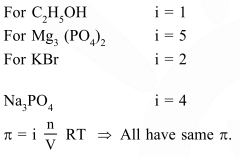Deepak Scored 45->99%ile with Bounce Back Crack Course. You can do it too!

# Consider separate solution of 0.500 M

Question:

Consider separate solution of $0.500 \mathrm{M} \mathrm{C}_{2} \mathrm{H}_{5} \mathrm{OH}(\mathrm{aq}), 0.100 \mathrm{M} \mathrm{Mg}_{3}\left(\mathrm{PO}_{4}\right)_{2}(\mathrm{aq}), 0.250 \mathrm{M} \mathrm{KBr}(\mathrm{aq})$ and $0.125 \mathrm{M} \mathrm{Na}_{3} \mathrm{PO}_{4}(\mathrm{aq})$ at $25^{\circ} \mathrm{C}$. Which statement is true about these solutions, assuming all salts to be strong electrolytes ?

1. $0.125 \mathrm{M} \mathrm{Na}_{3} \mathrm{PO}_{4}(\mathrm{aq})$ has the highest osmotic pressure.

2. $0.500 \mathrm{M} \mathrm{C}_{2} \mathrm{H}_{5} \mathrm{OH}(\mathrm{aq})$ has the highest osmotic pressure.

3.  They all have the same osmotic pressure

4. $0.100 \mathrm{M} \mathrm{Mg}_{3}\left(\mathrm{PO}_{4}\right)_{2}(\mathrm{aq})$ has the highest osmotic pressure.

Correct Option: , 3

Solution: